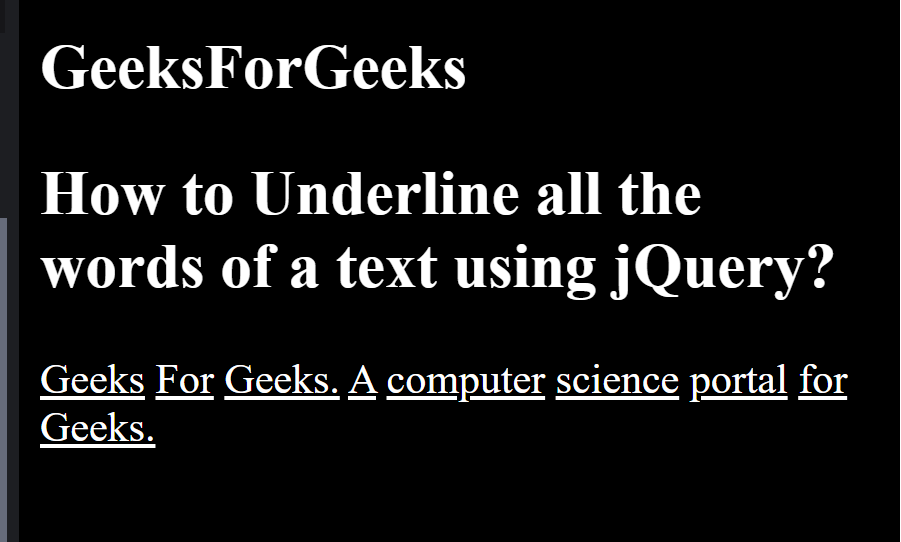# How to underline all words of a text using jQuery ?

Given a statement and the task is to underline all the text words of HTML page using jQuery. We will use text-decoration property to add underline to each words.

HTML code:

## HTML

 ` ` `<``html``> ` ` `  `<``head``> ` `    ``<``style``> ` `        ``p span { ` `            ``text-decoration: underline; ` `        ``} ` `    `` ` ` `  `    ``<``script` `src``= ` `"https://code.jquery.com/jquery-git.js"``> ` `    `` ` ` ` ` `  `<``body``> ` `    ``<``h2``>GeeksForGeeks ` `     `  `    ``<``h2``> ` `        ``How to underline all the words  ` `        ``of a text using jQuery? ` `    `` ` ` `  `    ``<``p``> ` `        ``Geeks For Geeks. A computer  ` `        ``science portal for Geeks. ` `    `` ` ` ` ` `  ` `

jQuery Code:

 `\$(``'p'``).each(``function` `() { ` ` `  `    ``var` `text_words = \$(``this``).text().split(``' '``); ` ` `  `    ``\$(``this``).empty().html(``function` `() { ` ` `  `        ``for` `(i = 0; i < text_words.length; i++) { ` `            ``if` `(i === 0) { ` `                ``\$(``this``).append(``''`  `                ``+ text_words[i] + ``''``); ` `            ``} ``else` `{ ` `                ``\$(``this``).append(``' '`  `                ``+ text_words[i] + ``''``); ` `            ``} ` `        ``} ` `    ``}); ` `}); `

Final code: The following code is the combination of the above two code snippets.

 ` ` `<``html``> ` ` `  `<``head``> ` `    ``<``style``> ` `        ``p span { ` `            ``text-decoration: underline; ` `        ``} ` `    `` ` ` `  `    ``<``script` `src``= ` `"https://code.jquery.com/jquery-git.js"``> ` `    `` ` ` ` ` `  `<``body``> ` `    ``<``h2``>GeeksForGeeks ` ` `  `    ``<``h2``> ` `        ``How to underline all the words  ` `        ``of a text using jQuery? ` `    `` ` ` `  `    ``<``p``> ` `        ``Geeks For Geeks. A computer  ` `        ``science portal for Geeks. ` `    `` ` `     `  `    ``<``script``> ` `        ``\$(document).ready(function () { ` `            ``\$('p').each(function () { ` `                ``var text_words =  ` `                    ``\$(this).text().split(' '); ` ` `  `                ``\$(this).empty().html(function () { ` `                    ``for (i = 0; i < ``text_words.length``; i++) { ` `                        ``if (i === 0) { ` `                            ``\$(this).append(''  ` `                            ``+ text_words[i] + ''); ` `                        ``} ` `                        ``else { ` `                            ``\$(this).append(' <``span``>'  ` `                            ``+ text_words[i] + ''); ` `                        ``} ` `                    ``} ` `                ``}); ` `            ``}); ` `        ``}); ` `    `` ` ` ` ` `  ` `

Output:Supported Browsers are listed below: